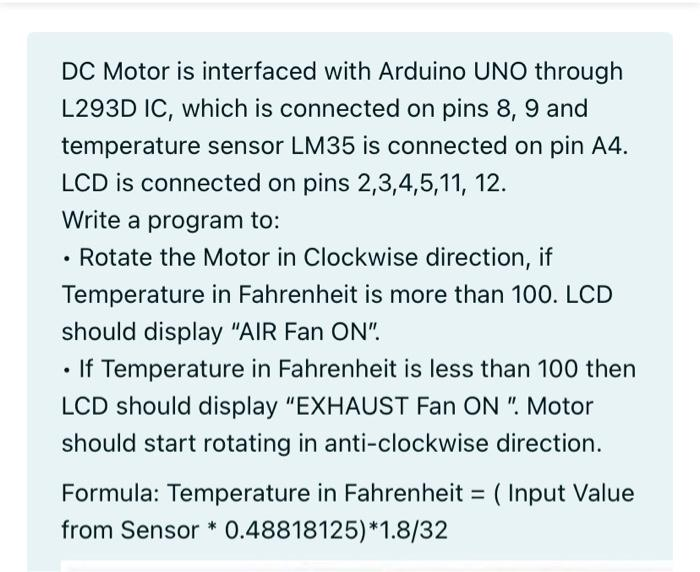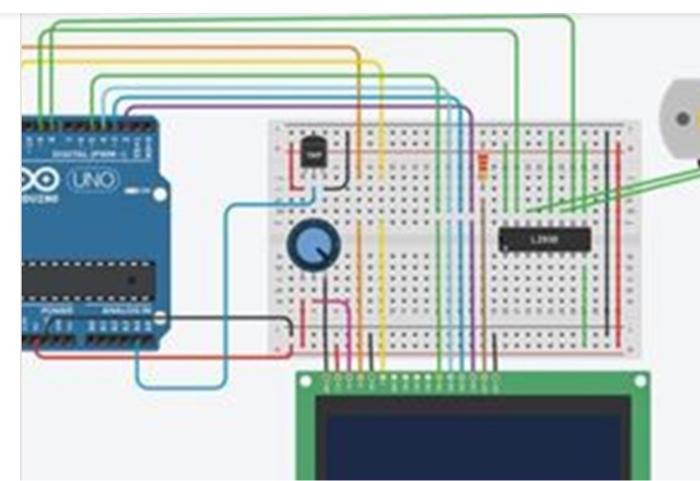# Question DC Motor is interfaced with Arduino UNO through L293D IC, which is connected on pins 8, 9 and temperature sensor LM35 is connected on pin A4. LCD is connected on pins 2,3,4,5,11, 12. Write a program to: • Rotate the Motor in Clockwise direction, if Temperature in Fahrenheit is more than 100. LCD should display "AIR Fan ON". • If Temperature in Fahrenheit is less than 100 then LCD should display "EXHAUST Fan ON ". Motor should start rotating in anti-clockwise direction. Formula: Temperature in Fahrenheit = ( Input Value from Sensor * 0.48818125)*1.8/32 DO UNO 0 ELEZJZ8U9 The Asker · Electrical EngineeringTranscribed Image Text: DC Motor is interfaced with Arduino UNO through L293D IC, which is connected on pins 8, 9 and temperature sensor LM35 is connected on pin A4. LCD is connected on pins 2,3,4,5,11, 12. Write a program to: • Rotate the Motor in Clockwise direction, if Temperature in Fahrenheit is more than 100. LCD should display "AIR Fan ON". • If Temperature in Fahrenheit is less than 100 then LCD should display "EXHAUST Fan ON ". Motor should start rotating in anti-clockwise direction. Formula: Temperature in Fahrenheit = ( Input Value from Sensor * 0.48818125)*1.8/32 DO UNO 0 ELE
More
Transcribed Image Text: DC Motor is interfaced with Arduino UNO through L293D IC, which is connected on pins 8, 9 and temperature sensor LM35 is connected on pin A4. LCD is connected on pins 2,3,4,5,11, 12. Write a program to: • Rotate the Motor in Clockwise direction, if Temperature in Fahrenheit is more than 100. LCD should display "AIR Fan ON". • If Temperature in Fahrenheit is less than 100 then LCD should display "EXHAUST Fan ON ". Motor should start rotating in anti-clockwise direction. Formula: Temperature in Fahrenheit = ( Input Value from Sensor * 0.48818125)*1.8/32 DO UNO 0 ELE